# What is a Resistor, Different types of resistors & Applications?

## Introduction:What is a Resistor, Different types of resistors & Applications- Resistors are found everywhere and are used in almost every electronic circuits, devices, and projects. There are many different types of resistors available in the market having different properties and used in so many different ways in different types of circuits. Due to its high demand every month millions of students and professionals, belonging to different fields of electrical and electronics engineering, search about the resistors to learn something that can be practically implemented while designing any electronic circuit.

When I was a student of engineering, the first electronic component, which I was introduced to in the Electronics Lab was a resistor. In the Lab, I learned some basic things like, how to find the resistance of a resistor with and without using a digital multimeter, and this was the time when I learned about the resistor color coding. Slowly and gradually I learned about how to use resistors in series and parallel and how to use the ohm’s law in practical circuits.

But before I write an article on how to use resistors in series and parallel and how to find the value of a resistor using the color codes, there are things that you need to know about. A resistor is not that simple as we think, we have so many different types of resistors which are used in different situations.

In this article, I will only explain the extreme basics like for example.

• What is a resistor?
• Resistor definition
• Resistor Units
• Resistor Symbol
• Different types of Resistors (Linear and Non-Linear)

Without any further delay, let’s get started!!!

## What is a Resistor?

No doubt, resistors are used in virtually all electronic circuits.

### Resistor Definition:

A resistor is a passive electronic component and is used to create resistance in the flow of electric current or in simple words it limits the flow of electrons through a circuit. I used the term passive which means that resistors only consume power and they cannot generate the Power.

What does a resistor do?

What is a resistor used for?

Why do we use a resistor?

These are of the type of questions which are most frequently asked by the beginners.

Resistors are most commonly used to limit the current, divide the voltage, and used as the Pull-Up or Pull-Down resistors with the microcontroller I/O pins.

### Resistor Units:

The resistance of a resistor is measured in Ohm’s which I am sure you already know about. A Greek symbol Omega “ Ω “ is used to represents the resistance. So, 1Ω “1 Ohm” can be defined as the resistance between two points where 1V of the applied voltage will push 1 ampere “1A” of current.

The smaller and larger resistor values are matched with the prefixes like Kilo ohm “KΩ, Mega ohm “MΩ”, or Giga ohm, etc.

For example, 1000 ohms can be written as 1kΩ, 10000 ohms can be written as 10kΩ, 4700 ohms can be written as 4.7kΩ, 1000000 = 1MΩ, and so on.

### Resistor Symbol:

A resistor symbol is also known as the schematic symbol. All resistors have two terminals except the variable resistors or some other special type of resistors which can have multiple legs. R1 is the American style 10KΩ and R2 is an international-style 1KΩ.

The American style symbol and International style symbol both are available in the Cadsoft Eagle schematic and PCB designing software. In fact, you can find these resistor symbols in all types of circuit designing software.For the easy understanding, every resistor in a circuit should have a unique name like R1, R2, R3, and so on. The same thing applies to all the electronic components. As in the programming we use unique variable names to easily differentiate one variable from the other. So, the same terminology is used in circuit designing as well.

## Types of Resistors:

As I said earlier resistors are available in different sizes, shapes, and materials. Different types of resistors can be clearly seen in the chart/tree given below. We will discuss the advantages and disadvantages of each type of resistor.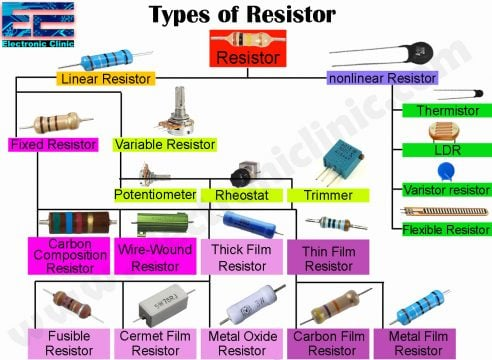There are mainly two types of resistors.

• Linear Resistors
• Non Linear Resistors

### Linear Resistors:

A linear resistor is one of the most commonly used resistors. The resistance of the linear resistor remains constant no matter if the potential difference or the voltage applied is increased or decreased. A linear resistor has a fixed resistance which does not change. A linear resistor has a further two types that have linear properties.

• Fixed Resistors
• Variable Resistors

#### Fixed Resistors:

The majority of the resistors used in the electronic circuits are of the type fixed resistors. The fixed resistors are of the type resistors which has the fixed resistance value. It is not possible to change/vary the resistance of the fixed resistor. A fixed resistor can be defined as the resistor whose resistance does not change with the change in voltage or temperature. Unlike the other types of resistors, fixed resistors are also available in various sizes and shapes.

Most commonly used fixed resistors are 1kΩ, 100Ω, 10kΩ, and 330Ω. These are of the type of resistors which I believe every beginner should have.

There are four types of Fixed Resistors.

• #### Thick Film Resistors

##### Carbon Composition Resistors: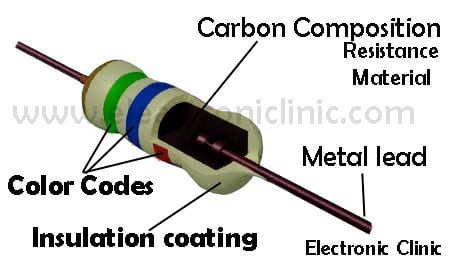The carbon composition resistors were the most frequently used resistors in the 1960s and earlier. But, due to its high cost and low stability, these resistors are nowadays rarely used.

The carbon composition resistor restricts the flow of current to a certain level. The Carbon composition resistors are made from the ceramic and the mixture of carbon powder.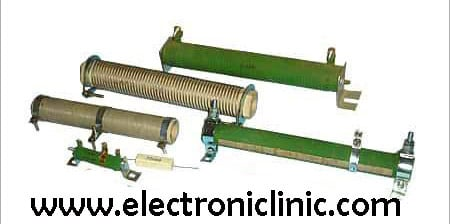##### Wire Wound Resistors:

The wire-wound resistors are made up by winding the metal wire around the insulating core or rod. The metal wire around the core acts as the resistive element which restricts the flow of electric current. This wire is made up of Tungsten, Manganin, Nichrome or nickel or nickel-chromium alloy. While the insulating core is made up of Bakelite, Porcelain, press bond paper, or ceramic clay material.

The wire-wound resistors in which the manganin is used are very costly and they are used with sensitive test equipment, for example, Wheatstone bridge, etc. These types of resistors can be safely operated in temperatures up to 350C. There are available from 2 watts up to 100-watt power rating or even more.

As compared to the carbon composition resistors the wire wound resistors make lower noise. The Performance of the wire-wound resistors is great in overload conditions.

The disadvantage of wire wound resistors:

The wire-wound resistors are very expensive and they cannot be used in high-frequency equipment.

Wire Wound Resistors application:

The wire-wound resistors are used where we need

• High sensitivity
• Accurate measurement and
• Balanced current control.

For example, it is used as the shunt resistor with ampere meters, the wire wound resistors are commonly used in high power rating devices and equipment, these are used in industries, and control equipment. The wire-wound resistors are the oldest type of resistors and have excellent properties. During their use, these resistors can become very hot, and for this reason, these are housed in a fnned metal case for heat dissipation.

##### Thick Film Resistors:

Thick film resistors are produced by applying a resistive film or paste, a mixture of glass and conductive materials, to a substrate. Thick film technology allows high resistance values to be printed on a cylindrical or flat substrate either covered entirely or in various patterns. The production technique of the thick film resistors is exactly the same as the thin film resistors; the only difference is that in thick film resistors we have a thick film instead of a thin film or layer of resistive material. There are three types of thick film resistors.

• Metal Oxide Resistors
• Cermet Film Resistors
• Fusible Resistors
###### Metal Oxide Resistors:

The Metal Oxide Resistors are fixed form, axial resistors. They are made of the ceramic rod that is coated with a thin film of metal oxides, such as tin oxide. These resistors are available in a wide range of resistance with high-temperature stability. These resistors can be used at high voltage and the level of operating noise is very low.

###### Properties of the Metal Oxide Film Resistors:

Metal oxide film resistors exceed the performance of metal film and carbon film for the following properties.

• Power Rating
• Voltage rating
• Surges and high temperatures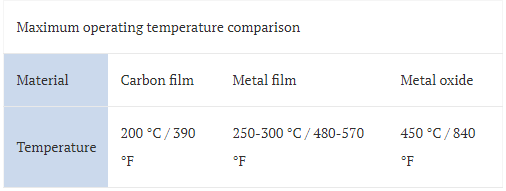##### Cermet Film Resistors “Network Resistors”:Cermet Film Resistors are a type of thick film resistor for which a thicker conducting paste is used. The paste is a mixture of both ceramic and metal. The Cermet film resistors possess qualities of low noise, good temperature stability, and decent voltage ratings. In the cermet film resistors, the internal area contains the ceramic insulation materials. The Cermet Film resistors are also called Network resistors are a combination of resistances that give identical value to all pins. These resistors are available in single inline and dual-inline packages.

##### Fusible Resistors:

Fusible resistors are just like the wire wound resistors. The fusible resistors are designed for certain power rating; when the power rating is increased than the specified value, then this resistor is fused, once fused i.e. it breaks or opens the circuit. So these resistors limit the current and can also be used as the fuse.

###### Application of Fusible resistors:
• Widely used in TV sets
• Amplifiers

The fusible resistors are most commonly used in an expensive electronic circuits. The ohmic value of the fusible resistors is less than 10 ohms.

### Variable resistors:These are the type of resistors in which the resistance can be changed manually by rotating the knob of the variable resistor. These type of resistors are used to set the reference voltage, there are also used in tuning circuits. These variable resistors are also used with the microcontroller’s analog pins. These resistors have mostly three legs, the rightmost and leftmost legs of the variable resistor are connected with the voltage and ground. We get the voltage on the middle leg of the variable resistor. This is nothing but a voltage divider.There are three types of variable resistors.

• Potentiometers
• Rheostats
• Trimmers

#### Potentiometers:

Normally, a Potentiometer is a three-legged electronic component which is used for controlling the voltage in a circuit. The resistance between the rightmost and leftmost legs is constant while the middle leg is connected with the moving part(wiper) which is variable. By changing the resistance which is done by rotating the knob of the Potentiometer we can get different voltages.

Inside the Potentiometer, there is a Carbon Track, Rotating wiper, a shaft, Resistive material, and terminals.

#### Rheostats:

A Rheostat is an electrical instrument used to control the current by varying the resistance. This is used for the current limiting purposes which are done by hand or manual operation. A Rheostat is usually two or three-terminal devices.

A Rheostat has a wire wound, adjustable tap, housing, and terminals.

The beginners are often confused with Rheostats and Potentiometers. Basically, there is no difference between Rheostat and Potentiometer, both are variable resistors. The main difference is in the use, for which purpose you are going to use this variable resistor? If you are using it for controlling the level of voltage then this variable resistor would be called as the potentiometer otherwise a Rheostat.

#### Trimmers:

Trimmer potentiometers are sometimes referred to as trim pots or trimmer resistors are a type of adjustable potentiometer (variable resistor). They are used to calibrate and fine-tune circuits. The trimmers are usually constructed from cermet or have Carbon Composition.

### Non-Linear Resistors:

I personally love this category of resistors. I have been using Non-Linear Resistors for such a long time. Nonlinear resistors are those types of resistors, in which the flow of current changes with change in temperature or the applied voltage.

There are three types of Non-Linear Resistors.

• Thermistors
• Varistors(VDR)
• A photoresistor or photoconductive cell (LDR)
• Flexible Resistor

Thermister is also a resistor in which the current flowing through a resistor changes with change in the temperature.

A varistor is a type of resistor in which the current flow changes with the applied voltage, so the current flow can be changed by changing the applied voltage. A varistor is also called VDR(Voltage Dependent Resistor).

Photo Resistor or Photo Conductive Cell (LDR), is a type of resistor in which the current flow changes with the amount of light following the device. This is a variable resistor and the resistance changes with the amount of light falling on the sensors. Most of the nonlinear resistor is used as the Sensors and are connected with the analog pins of the controllers.

Flexible Resistor which is also known as the flex sensor or bend sensor is a sensor that measures the amount of bending or deflection. The Flex sensors are used for the precise angle measurement. I have a very detailed tutorial on this sensor which you can find the related projects section given below.

## Resistors application:

All the types of resistors so for discussed are used for the following purposes.

• For current controlling and limiting
• As a multiplier in a voltmeter.
• As the voltage dividers
• To change electrical energy in the form of heat energy
• To control voltage or drop
• As a shunt in Ampere meters
• For protection purposes, e.g, Fusible resistors
• Widely used in the electronics industries
• Used as sensors with microcontrollers
• In-home electrical appliances like heater, iron, immersion rod etc.

## Want to Know more about Resistors and Suppliers?

### MOV resistor:

MOV stands for Metal Oxide Varistor. MOV resistor is a type of resistor whose resistance changes with the applied voltage. This is a nonlinear resistor and provides excellent transient voltage compression. The MOV resistor or Metal Oxide Varistor is designed to protect various types of electronic devices and semiconductor elements from switching and induced lightning surges.

### Zero-ohm Resistors, Or resistors:

It is quite clear from the name Zero-ohm Resistors, these are of the type resistors which has almost no resistance at all. In reality this resistance is not 100% zero, but its near to 0 ohms. The Zero-ohm resistors offer very small resistance. These resistors are available in 2/4W and 1/8W power ratings.

### Why use Zero-ohm Resistors?

The first question which pops up in everyone’s mind is, why we need a resistor which has a resistance equal to zero? Rather than using a zero-ohm resistor why not use a normal jumper wire.

The zero-ohm resistors are used in PCB manufacturing companies as you know today the components in printed circuit boards are inserted by automatic insertion machines to speed up the manufacturing process rather than manually by humans. There are situations when two points need to be short on the printed circuit board, the automatic machines in use can only handle components such as resistors but not jumper wires. For jumper wires a separate machine may be needed or these wires should be manually inserted by humans. So, instead zero-ohm resistors are used.

The main advantage of the zero-ohm resistors is that, these resistors can be easily removed and can be easily replaced with other resistor if needed.

### SMD Resistors (Surface Mounted Device):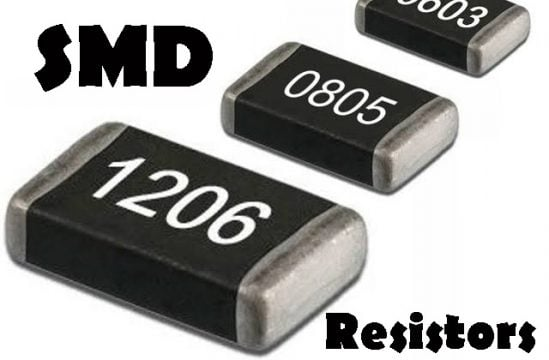SMD stands for Surface Mounted Device. An SMD is an electronic component that is made to use with SMT, or Surface Mount Technology. The SMD resistors are easy to install by the Automatic machines than the regular resistors. The SMD resistors can be easily installed and removed. The SMD technology helped the PCB manufacturing companies to speedup the manufacturing process. This is just because of the SMD technology the sizes of the electronic devices reduced and also the costs are reduced.

### Resistor switch:

Is a resistor a Switch? This is one of the most frequently asked questions. People are searching about this. Well, Resistor is not a switch, it is an electronic component that resists the flow of current or controls the flow of current. But a resistor can be used with a button as the Pullup resistor or as the pulldown resistor, which gives the microcontroller a signal. Which can be a 5v signal or a 0”Gnd”.

Read my article on Push Button Switch wiring and code “https://www.electroniclinic.com/arduino-push-button-switch-wiring-and-code-beginners-level/

### Blue Resistor:

Blue-colored body of a resistors indicates a tolerance of 1% or 2%. Blue color resistors contain Metal Oxide film elements.

### Green Resistor:

The Green Color resistors contain Carbon Film.

### Equivalent resistor:

You know about the Series circuits and parallel circuits where we calculate the equivalent resistance, and then replace that with just a single resistor. Let’s say you have 4 resistors connected in parallel and let’s say 3 resistors in series. In a circuit like this you can find the equivalent resistance, and replace those 4 resistors with just 1 equivalent resistor.

### High voltage resistor:

High Voltage Resistors are one of the most commonly used resistors in office equipment and home appliances,

Copying Machines

Printers

Air-conditioners etc.

The High Voltage resistors offers high resistance and small deviation values.

NTC resistor:

NTC stands for “Negative Temperature Coefficient”. The NTC thermistors or resistors are the nonlinear resistors with a negative temperature coefficient, which means that the resistance decreases with increasing temperature. The NTC resistors or Sensors are typically used in a range from -55C to 200C.

## Resistors suppliers & Manufacturers:

### State of the art resistors:

State of the Art, Inc deals in

• Chip Resistors
• Precision Chip Resistors
• Military Products
• High Frequency Products
• Resistor Networks
• Special Applications.

Website: http://www.resistor.com/

### RCD Components, Inc.

RCD Resistors

RCD (Resistors Coils Delaylines) Resistors